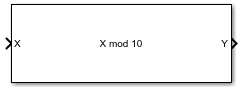# Modulo by Constant

Perform modulo operation with a constant denominator

• Library:
• Fixed-Point Designer

•## Description

The Modulo by Constant block performs the modulo operation (remainder after division) with a constant denominator.

The Modulo by Constant block uses an algorithm that is functionally similar to a Barrett Reduction. The division operation is computed via a multiplication by inverse, which generally results in better performance on embedded systems.

## Ports

### Input

expand all

Dividend, specified as a real scalar.

If X is a fixed-point data type, it must use binary-point scaling.

Data Types: `single` | `double` | `int8` | `int16` | `int32` | `int64` | `uint8` | `uint16` | `uint32` | `fixed point`

### Output

expand all

Result of modulus operation, returned as a scalar.

Data Types: `single` | `double` | `int8` | `int16` | `int32` | `int64` | `uint8` | `uint16` | `uint32` | `fixed point`

## Parameters

expand all

Divisor to use for the modulus operation, specified as a positive, real-valued, finite scalar.

#### Programmatic Use

 Block Parameter: `Denominator` Type: character vector Values: MATLAB® expression that evaluates to a positive, real-valued, finite fixed point or numeric value Default: `'10'`

Data Types: `single` | `double` | `int8` | `int16` | `int32` | `int64` | `uint8` | `uint16` | `uint32` | `uint64` | `Boolean` | `fixed point`

## Extended Capabilities